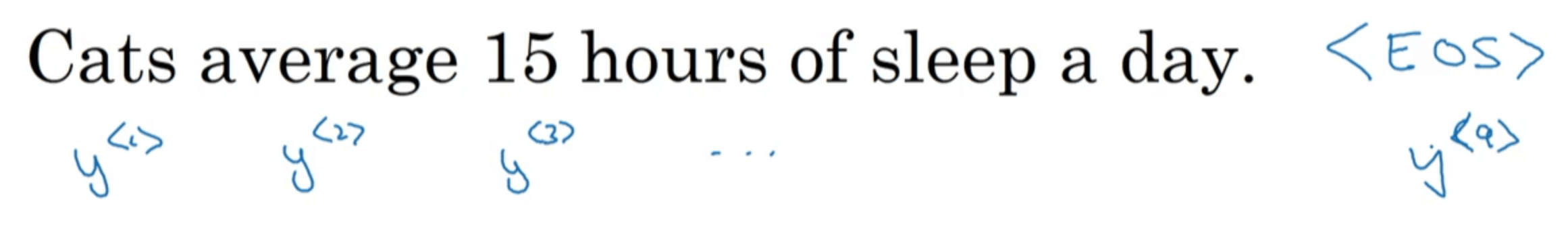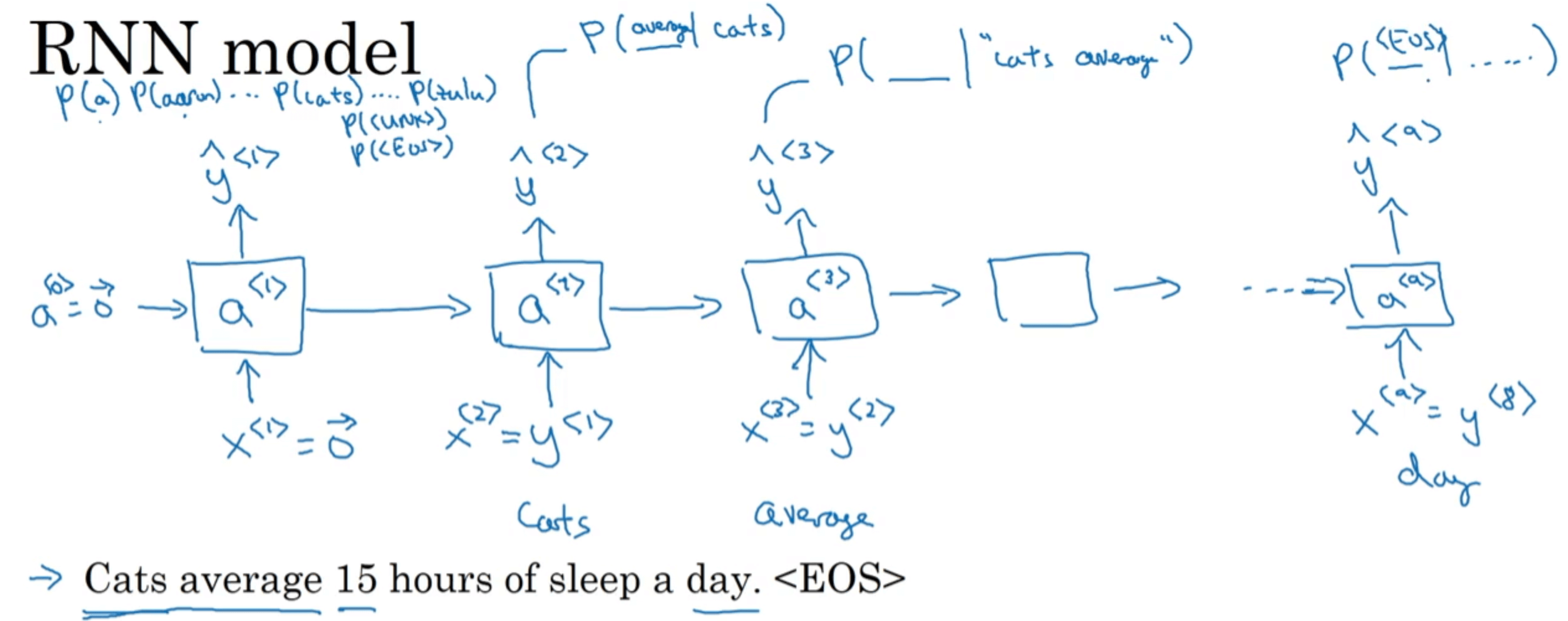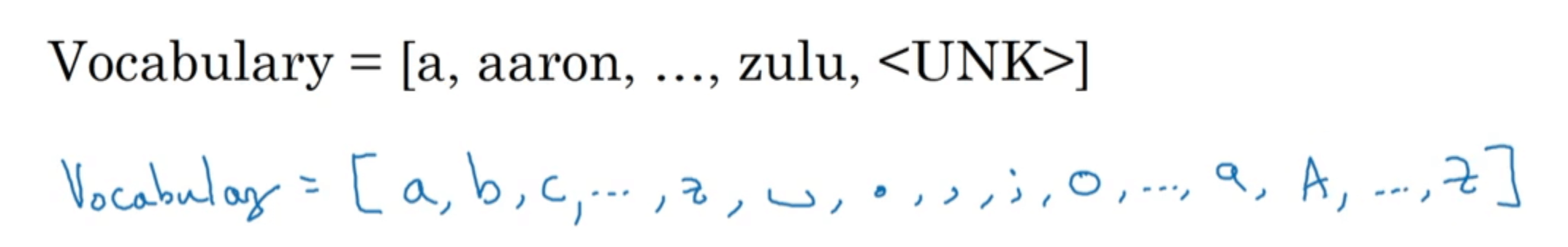# 【深度学习基础】第四十一课：RNN应用之语言模型

## 语言模型

Posted by x-jeff on November 30, 2020

【深度学习基础】系列博客为学习Coursera上吴恩达深度学习课程所做的课程笔记。

# 2.如何使用RNN构建语言模型$x^{<1>}$和$a^{<0>}$均初始化为零向量。然后通过softmax函数预测出第一个单词最可能是哪个，在本例中为单词“Cats”。并且将$\hat{y} ^{<1>}$（也就是$x^{<2>}$）作为输入传给下一步。然后同样通过softmax函数预测出第二个单词最可能是哪个。也就是说在预测第二个单词时，会利用第一个单词为“Cats”这一信息，类似于条件概率$P(第二个单词｜”Cats”)$。剩余的以此类推。

cost function可定义如下：

$L^{<t>}(\hat{y}^{<t>},y^{<t>})=-\sum_i y_i^{<t>} \log \hat{y}_i ^{<t>}$ $L=\sum_t L^{<t>}(\hat{y}^{<t>},y^{<t>})$

$i$为所用字典中的第$i$个单词。• 优点：
1. 不会出现UNK
• 缺点：
1. 序列会很长。
2. 不善于捕捉句子中单词之间的依赖关系。
3. 计算成本较高。